Next: A generalization for the Up: DECONVOLUTION WITH A KNOWN Previous: The basic operator

## Building a more complex operator

Both low- and high-frequency components of the reflectivity series will not be correctly resolved by the iterative process described by equation (6). When two adjacent reflectivities with the same polarity are sufficiently close, they will not be represented by distinguishable peaks in the correlation series. The method will see" a single reflectivity, with an amplitude close to the sum of the amplitudes of the two actual reflectivities. Similarly, if two close, adjacent reflectivities have opposite polarity, the scaled correlation will still show two peaks but with amplitudes smaller than the amplitudes of the original reflectivities.

One way to avoid the excessive influence of the initial picking steps (which is crucial to retrieve the lower-frequency components of the reflectivity) is to introduce a damping factor in equation (6)(7)

Although fast on the beginning, the convergence rate tends slow after the initial iterations. To overcome this problem I use a least-squares inversion of the residuals after a specified number of iterations of the method. I formulate the least-squares problem so that the objective function to be minimized is(8)

where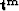are the residuals of the last iteration of the method, and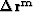are the unknowns. The final estimated reflectivities will be given by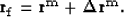(9)

As suggested by Claerbout (1989), to generate a sparse synthetic sequence, we can take an integer power of a series comprised of Gaussian noise. I used the third power to build a synthetic reflectivity sequence, because it represents about the limit of resolution for wavelet estimation (for a white-spectrum wavelet). Figures 2 and 3 show a comparison between different approaches used to deconvolve the synthetic trace.

We can see from Figure 2 that when the basic operator is used (c) most of the major events are correctly recovered, but the low-frequency components of the reflectivities are not properly resolved (e.g., the negative event between 0.3 and 0.4 seconds). When a damping factor of 0.5 is introduced (d) the low-frequency components of the original reflectivity are better retrieved but the high-frequency components are still unresolved, as we can see by the events beyond 0.6 seconds. These events are better resolved in the last trace of this figure (e), which refers to the least-squares residual inversion applied to (d).knowt1
Figure 2
(a) the synthetic trace generated by the convolution of the wavelet (a minimum-phase Ricker) with the synthetic reflectivity series (b), obtained by taking the third power of a Gaussian noise sequence. The other three traces correspond to the reflectivities retrieved by different versions of the iterative correlation-picking decon. (c) corresponds to the basic operator described by equation (6); in (d) a damping factor of 0.5 was introduced according to equation (7); and in (e) an extra residual-least-squares step is applied on the output of the damped operator as in equation (9). All cases correspond to 250 iterations, which is the number of samples.Figure 3 shows a comparison between the reflectivity series retrieved by different methods: predictive deconvolution in (b), convolution with the least-squares inverse of the wavelet in (c), direct least-squares inversion in (d), and the least-squares applied to the residuals of the damped, iterative correlation-picking decon (e) , which is the same as (e) in Figure 2. The direct least-squares and the correlation-picking deconvolutions correspond to the sequences that better fit the original series (a). It is clear, however, that the iterative correlation-picking (ICP) result has the same frequency content as the original sequence, while the least-squares inversion (LSI) corresponds to its lower-frequency version.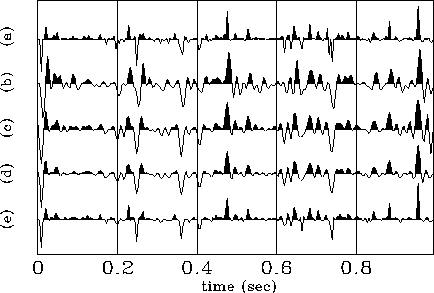knowt2
Figure 3
(a) corresponds to the original (true) reflectivity sequence (as Figure 2-b); the other traces are the reflectivity series retrieved by different algorithms: (b) is the result of the predictive deconvolution (plotted with a scale factor of 13); while in (c) a least-squares inversion was performed for the known wavelet and the resulting inverse filter convolved with the original trace (Figure 2-a). (d) corresponds to the result of the direct least-squares inversion of the original trace (plotted with a scale factor of 1.45), and (e) is the reflectivity series retrieved by the ICP algorithm with a residual least-squares step (as in Figure 2-e).The spectra of the synthetic trace, the original reflectivity series, and the reflectivities recovered by each of the methods described in Figure 3, are shown in Figure 4. In the central frequency range of the original wavelet (10-50 Hz) all methods, except the predictive decon, correctly recover the shape of the reflectivity spectrum. Since the predictive decon does not use any information regarding the original wavelet (except that it is minimum-phase), its relative weakness compared to other methods would be expected. In the low-frequency region however, only the ICP and LSI algorithms properly retrieve the shape of the original reflectivities. All methods, except the ICP, show a decay for high frequencies that follows the same decay pattern of the trace (and wavelet) spectrum. That is why, although with the same shape, the spectra retrieved by those methods have a higher amplitude than the spectrum of the true reflectivities in the low and medium range of frequency.

The ICP algorithm, however, keeps the same amplitude level in the whole frequency domain, with two direct consequences: it exactly recovers both the shape and the amplitude of the original spectrum in the range of frequencies covered by the wavelet, and it loosely recovers the part of the spectrum that is not covered by the wavelet. This result is better than just zero this part of the spectrum.knows
Figure 4
In all graphs the dashed line corresponds to the spectrum of the original trace, the continuous line corresponds to the spectrum of the original reflectivity sequence, and the dotted line applies for the spectra of the retrieved reflectivity sequences of Figure 3 b-e. (a) predictive decon, (b) convolution with least-squares inverse wavelet filter, (c) LSI algorithm, and (d) ICP with residual least-squares step.Final comparisons between the LSI and ICP algorithms are presented in Figures 5 and 6. In the first of these figures, I show that when the ICP algorithm with a residual least-squares step is used, the residuals () are smaller than those of the LSI algorithm. In Figure 6 I compare the impedance profiles obtained from these two algorithms with the impedance obtained from the original reflectivity series. The impedance obtained with the ICP method shows a slightly better fitting of the true impedance, than the one obtained with the LSI method.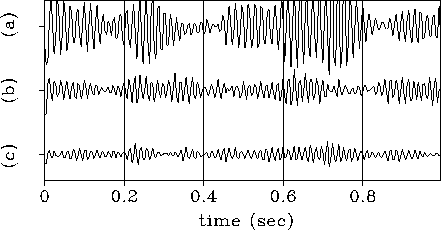knowdif
Figure 5
(a) Residuals of the damped ICP algorithm after 25,000 iterations, (b) residuals of the LSI algorithm, and (c) residuals of the damped ICP with residual least-squares algorithm after 250 iterations.imp
Figure 6
The continuous line is the true impedance obtained from the original synthetic reflectivity series, while the dashed and dotted lines correspond to the impedance obtained from the reflectivities retrieved by the LSI and ICP algorithms respectively.Next: A generalization for the Up: DECONVOLUTION WITH A KNOWN Previous: The basic operator
Stanford Exploration Project
1/13/1998